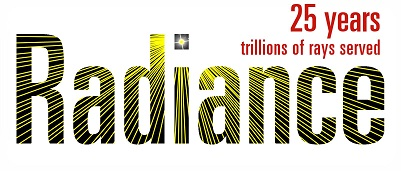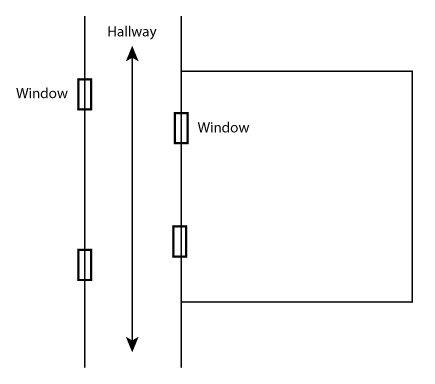# Multi-phase simulation through hallway

Hi all,

I’m interested in evaluating the daylight in the square room in the attached figure. There is an adjacent internal hallway. BSDFs are to be used on all four windows. From the tutorial by @sarith, I know how to evaluate the daylight if there is only one set of BSDFs, as if the exterior facade of the hallway was removed completely.

How would one go about simulating the daylight passing through the two sets of windows using BSDFs? If it is possible using multi-phase workflow with BSDFs?Hi @mikkelkp, the approach shown below might work.

A somewhat similar example is covered in section 8.8 of the tutorial. The commands for that example can be found in the tutorial files (I am linking the commands here). The main difference between that example and your model is that your model has an additional layer of transmission matrices.

You can test if this works or not by using a clear BSDF for the multi-phase simulation and then comparing the results with a daylight-coefficient simulation. If this is not a research project, you are probably better off sticking to a daylight coefficient simulation. If you have the Radiance model for that sketch, I can try running the simulation and get back to you in a few days.

another option would be to use a standard 3(or5)-phase method on the inner windows except to use equally high rendering parameters for the window->sky matrix, this matrix is often calculated with a reduced number of ambient bounces, but in this case you would need a full accounting of the indirect contribution. If the outer layer is clear glass, you would be better off using the glass modifier under this approach. To include dynamic controls, you would run this window->sky matrix once for each state and swap this matrix rather than the BSDF in the rmtxop -> illum.mtx step. This should not be a huge calculation burden, since you are only calculating this window->sky matrix for two points (one for each window) and unless the two windows are controlled differently with dynamic shading, you do not need to cross multiply the contributions the way you do with an additional phase.

Thanks for your suggestions both of you. So far I have tried the first one, though with one modification, so that step 1 is `R11 = F11 * To1 * D1, R12 = F12 * To2 * D2` and so on, eventually excluding the creation of `D1temp`. I tried an image-based simulation using Accelerad. The Radiance model along with the simulation can be found here (Dropbox).

I have not have the time yet to also do an illuminance simulation with sensor points as well as a DC simulation for comparison.